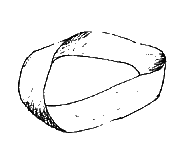## Quiz Solutions

Each quiz is out of 3 points. Your 3 worst quiz grades are dropped at the end of term.

#### Quiz #14 (26 Apr)

1. Construct a NFA using the mechanical method for `a(b|c)*|b*`.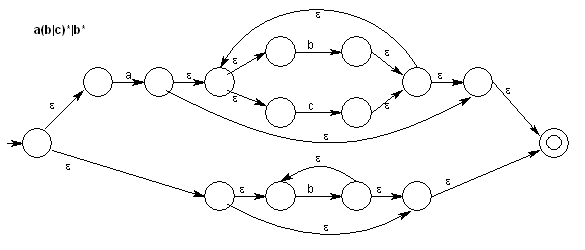2. Construct a minimal-state DFA equivalent to the following DFA: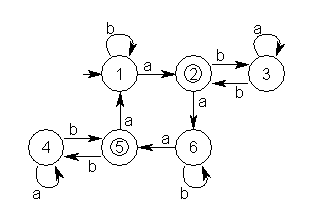```  NON-ACCEPT     ACCEPT
1,3,4,6        2,5
a)  /    \        /
3,4    1,6    2,5
b)  \     |      |
3,4   1,6    2,5
a)  /     \      |
3,4     1,6   2,5
b) \       |    /
3,4    1,6  2,5
```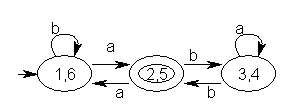#### Quiz #13 (24 Apr)

Use the mechanical method of construction to produce a NFA for `(a|b|c)+`.

Following a purely mechanical approach using only the seven building blocks given in lecture would produce this: ((a|b)|c)((a|b)|c)*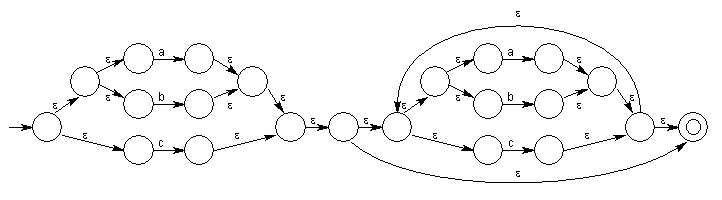However, if we had a building block for a 3-way "or" (R|S|T) and a "+" closure, we could produce this directly: (a|b|c)+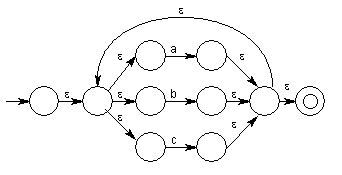#### Quiz #12 (10 Apr)

Produce a parse of `a*a*a+a` using the parsing machine [as given in the Set 24 notes].

```    SYMBOL   STATE       INPUT    TRANSITION/REDUCTION
1.  [empty]  0         a*a*a+a|   Initial state
2.  a        0 4        *a*a+a|   "a" trans from 0 to 4
3.  T        0 3        *a*a+a|   T->a reduction
4.  T *      0 3 5       a*a+a|   "*" trans from 3 to 5
5.  T * a    0 3 5 7      *a+a|   "a" trans from 5 to 7
6.  T        0 3          *a+a|   T->T+a reduction
7.  T *      0 3 5         a+a|   "*" trans from 3 to 5
8.  T * a    0 3 5 7        +a|   "a" trans from 5 to 7
9.  T        0 3            +a|   T->T*a reduction
10. E        0 1            +a|   E->T reduction
11. E +      0 1 2           a|   "+" trans from 1 to 2
12. E + a    0 1 2 4          |   "a" trans from 2 to 4
13. E + T    0 1 2 6          |   T->a reduction
14. E        0 1              |   E->E+T reduction
15. ACCEPT

("|" = end of file)
```

#### Quiz #11 (22 Feb)

1) (OPEN BOOK) Provide the code required to produce the output shown from clock 1: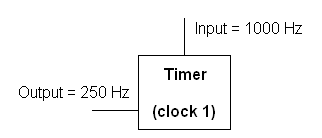```; program clock 1 to produce a 250 hz signal (following example code format)
mov	al, 01110110b	;initialize timer clock 1
out	43h, al
mov	ax, 4		;divide 1000hz input by 4 to get 250hz output
out	41h, al		;clock 1 on port 41h, send low byte of divisor
mov	al, ah
out	41h, al		;send high byte of the divisor
```

2) (CLOSED BOOK) Describe the set of signals that occur when you press a key and how the interrupt routine for the keyboard gets executed: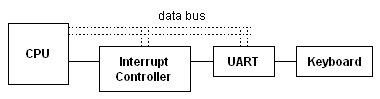```Key pressed goes to UART
UART signals interrupt controller
IC interrupts CPU
(if current routine can be interrupted by keyboard),
specifying that a keyboard interrupt occurred
CPU finishes current instruction,
and then saves state (next instruction and flags)
CPU calls system subroutine for handling keyboard input
CPU/Subroutine acknowledges IC
CPU reads data from UART along data bus
CPU returns to stored next instruction
```

#### Quiz #10 (20 Feb)

Assuming graphics mode 13h, determine the COL and ROW of the point indicated (front of top/right tail gun). (Image not to scale.)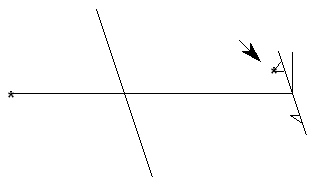Nose (point): COL 200, ROW 100
Body (length): 50 pixels
Tail wing (diagonal length): 40 pixels (20 each side)
Gun: triangle with horizontal base of 7 pixels, attached 5 pixels from the body.

```Since we know the location of nose, we just determine the offset from there.

COL/x = 200 (nose) + 50 (body) - 5 (tail wing) - 7 (gun base) = 238
ROW/y = 100 (nose) +  0 (body) - 5 (tail wing) - 0 (gun base) = 95

```

#### Quiz #9 (15 Feb)

Write the code to draw, in graphics mode 13h, a horizontal line of length 10 pixels, colored red (color code: 04h) whose left-hand end is at COL 40, ROW 60 of the graphics screen.

```	mov	ax, 0A000h
mov	es, ax		;so di now points into video memory

mov	ah, 00h		;get into graphics video mode
mov	al, 13h
int	10h

mov	di, (60 * 320) + 40	;row 60, col 40 (indexed from 0)
mov	al, 04h			;red pixel
mov	cx, 10			;line of length of 10 pixels
cld				;draw forward (left to right)
rep 	stosb
```

#### Quiz #8 (13 Feb)

Color codes: Yellow is Eh, red is 4h. Write a red 'D' on a yellow background at COL 32, ROW 9 by writing directly into video memory (ie, not using the `int` instruction). Text-based video memory starts at 80000h B800h.

```	mov	ax, 0B800h
mov	es, ax		;so di now points into video memory

mov	ah, 00h		;get into text video mode (optional)
mov	al, 03h		;(also clears screen)
int	10h

mov	di, 2 * ((9 * 80) + (32))	;row 9, col 32  (if indexed from 0)
mov	ah, 0E4h			;yellow backround, red foreground
mov	al, 'D'				;letter D
stosw
```

#### Quiz #7 (01 Feb)

Write a macro called `COMP` such that `COMP X, Y, N` compares the first N bytes of string X and Y, and sets AX = 1 if they are equal or AX = 0 if they are not equal. No checking needs to be made as to whether the lengths of X and Y are <= N or that X and Y are strings. Example of use: `COMP MES1, MES2, 5`

```	;Compares the first N characters of 2 strings (X and Y) for equality.
;If equal, sets AX = 1; else AX = 0.
;Does not preserve registers, so will also trash: ES, SI, DI, CX, and flags
COMP	MACRO X, Y, N
LOCAL MATCH, DONE		;to avoid duplicate label error
;if macro is used more than once
push	ds
pop	es			;so di in same segment as si

lea	si, X
lea	di, Y
mov	cx, N
cld				;ensure we'll go forwards
repe	cmpsb

jcxz	MATCH			;cx==0, so matched all of N chars
mov	ax, 0			;else...
jmp	DONE
MATCH:	mov	ax, 1
DONE:
ENDM COMP
```

#### Quiz #6 (30 Jan)

Assuming:

```	.DATA
M	DW	?
N	DW	?
...
.CODE
Main	PROC
...
push M
push N
...
Main	ENDP
```

Write the subroutine `ADDER` to set AX = M + N. Use the standard subroutine form.

```Adder	PROC
push	bp
mov	bp, sp
mov	ax, [bp + 6]		;M
add	ax, [bp + 4]		;N
pop	bp
ret	4
```

#### Quiz #5 (25 Jan)

Write the code to evalutate (M*N*T*S)/(R*F*G*H), assuming these are all pre-initialized word variables.

```	mov	ax, M
imul	N		;answer now in DX:AX
idiv	R		;now in AX
imul	T
idiv	F
imul	S
idiv	G		;now in AX, but remainder in DX
mov	DX, 0		;clear DX
idiv	H		;final answer in AX
```

#### Quiz #4 (23 Jan)

```  LIST 	DW 	10 DUP (?)
```

Assuming the 10 values in `LIST` have been initialized, write the code to add up the members of `LIST` with the result in `ax`. Use the `loop` instruction.

Here is one possible solution:

```	mov	ax, 0		;clear ax for addition
mov	bx, 0		;use a base pointer for offset into array
mov	cx, 10          ;loop 10 times
add	ax, LIST[bx]	;LIST[bx] is same as [LIST + bx]
add	bx, 2		;move to next word
;sum now in ax
```

#### Quiz #3 (18 Jan)

NUM DW ?

For each, identify the type of the second operand:

```MOV	AX, BX		;register
MOV	AX, NUM		;direct (memory)
MOV	AX, 32H		;immediate (constant)
MOV	AX, "IC"	;immediate (constant)
MOV	AX, [BX]	;indirect (memory)
```

#### Quiz #2 (16 Jan)

Write the outline code of an assembler program.

```	.MODEL small
.586		; allows Pentium instructions to be used
.STACK 100H

.DATA
; put data definitions here

.CODE
MAIN  	PROC
mov	ax, @data	;(optional: load ds seg register)
mov	ds, ax
;... procedure code here
mov	ax, 4C00h	;(optional: quit to Dos at end)
int	21h
MAIN	ENDP

SUB1  	PROC
;... a second procedure
SUB1  	ENDP

END MAIN
```

#### Quiz #1b (11 Jan -- Speed Test) [1 point]

```1) 6 = 0110b
9 = 1001b
Ah = 1010b
Dh = 1101b

2) 2^5 = 32
2^8 = 256
2^3 = 8
2^11 = 2,048

3) 1110b = Eh
0101b = 5h
1011b = Bh
1100b = Ch
```

#### Quiz #1a (11 Jan) [2 points]

```1) 2^9 = 512

2) 257,982 = Binary: 0011 1110 1111 1011 1110b
Hex:    3EFBEh

3) 0 1001 1010 1011 = Hex: 9ABh
Decimal: 2,475

4)  10 1101 1000
+ 00 1110 0110
11 1011 1110

5)  2FD03Fh
+ 3EE247h
6EB286h
```

 ~ztomasze Index : TA Details : ICS312 : Quiz Solutions http://www2.hawaii.edu/~ztomasze Last Edited: 07 May 2007 ©2006 by Z. Tomaszewski.